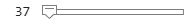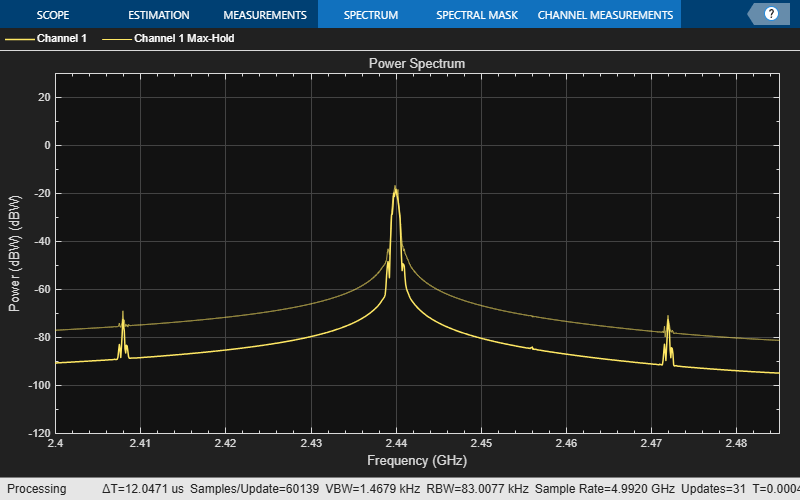# BLE Output Power and In-Band Emissions Test Measurements

This example shows how to perform transmitter test measurements specific to output power and in-band emissions on Bluetooth® Low Energy (BLE) transmitted waveforms as per the Bluetooth RF-PHY Test Specifications [ 1 ] using Communications Toolbox™ Library for the Bluetooth® Protocol.

### Objectives of BLE RF-PHY tests

The Bluetooth RF-PHY Test Specifications [ 1 ] defined by Bluetooth Special Interest Group (SIG) includes RF-PHY tests for both transmitter and receiver. The objectives of these RF-PHY tests are to ensure interoperability between all BLE devices and to verify that a basic level of system performance is guaranteed for all BLE products. Each test case has a specified test procedure and an expected outcome, which must be met by the implementation under test (IUT).

### RF-PHY Transmitter Tests

This example performs output power and in-band emissions test measurements according to the Bluetooth RF-PHY Test Specifications [ 1 ]. The output power measurement is designed to ensure that power levels are high enough to maintain interoperability with other Bluetooth devices and low enough to minimize interference within the ISM band. The in-band emission test is to verify that the level of unwanted signals within the frequency range from the transmitter do not exceed the specified limits. The test case IDs corresponding to the tests considered in this example are as follows:

Output Power:

RF-PHY/TRM/BV-01-C: This test verifies the maximum peak and average power emitted from the IUT are within limits.

In-band Emissions:

• RF-PHY/TRM/BV-03-C: This test verifies that the in-band emissions are within limits when the transmitter is operating with uncoded data at 1 Ms/s.

• RF-PHY/TRM/BV-08-C: This test verifies that the in-band emissions are within limits when the transmitter is operating with uncoded data at 2 Ms/s.

### Check for Support Package Installation

```% Check if the 'Communications Toolbox Library for the Bluetooth Protocol' % support package is installed or not. commSupportPackageCheck('BLUETOOTH');```

### Configure the Test Parameters

You can change `phyMode`, `Fc`, `outputPower` and `numDominantFreq` parameters based on the PHY transmission mode, frequency of operation, output power and number of dominant frequencies, respectively.

```% Select PHY transmission mode {'LE1M','LE2M'} as per Bluetooth RF-PHY Test % Specifications phyMode ="LE1M"; % Select frequency of operation for IUT based on the generic access profile % (GAP) role(s) as shown in the table below. % -------------------------------------------------------------------------------- % | Operating | Peripheral & Central Devices | Broadcaster & Observer Devices | % | Frequency | | | % | (MHz) |----------------------------------|---------------------------------| % | | Output Power | In-band Emissions | Output Power | In-band Emissions| % | | Test | Test | Test | Test | % |-----------|--------------|-------------------|--------------|------------------| % | Lowest | 2402 | 2406 | 2402 | 2402 | % | Middle | 2440 | 2440 | 2426 | 2440 | % | Highest | 2480 | 2476 | 2480 | 2480 | % -------------------------------------------------------------------------------- Fc = 2440e6; % Frequency of operation in Hz payloadLength =37; % Payload length in bytes, must be in the range [37,255] sps = 32; % Number of samples per symbol, minimum of 32 sps as per the test specifications outputPower =20; % Output power in dBm, must be in the range [-20,20] numDominantFreq =6; % Select number of dominant frequencies for in-band emissions test, must % be in the range [1,78] and [1,74] for LE1M and LE2M modes, respectively. % The number of dominant frequencies represents the number of test % frequencies near the operating frequency at which the in-band emissions % test is to be performed. The number chosen in this example leads to a % short simulation. For performing complete in-band emissions test, change % the |numDominantFreq| parameter to maximum number of dominant frequencies % as specified in the Section 4.4.2 of the Bluetooth RF-PHY Test % Specifications.```

### Generate BLE Test Waveforms

The function, helperBLETestWaveform.m, is configured to generate a BLE test waveform as per the Bluetooth specifications [ 2 ].

```payloadType = 0; % Payload type for PRBS9 sequence waveform = helperBLETestWaveform(payloadType,payloadLength,sps,phyMode); % Calculate sampling rate in Hz based on PHY transmission mode Rsym = 1e6; if strcmp(phyMode,'LE2M') Rsym = 2e6; end Fs = Rsym*sps; % Apply frequency upconversion to obtain a passband signal for the % specified frequency of operation. maxFreq = 2485e6; % in Hz interpFactor = ceil(2*maxFreq/Fs); % Interpolation factor for upconversion to % cover BLE RF frequency band (2400e6 to 2485e6) % Change the stopband frequency in Hz based on the PHY transmission mode stopbandFreq = 2e6; if strcmp(phyMode,'LE2M') stopbandFreq = 4e6; end % Create a digital upconverter System object upConv = dsp.DigitalUpConverter(... 'InterpolationFactor', interpFactor,... 'SampleRate', Fs,... 'Bandwidth', 2e6,... 'StopbandAttenuation', 44,... 'PassbandRipple',0.5,... 'CenterFrequency',Fc,... 'StopbandFrequencySource','Property',... 'StopbandFrequency', stopbandFreq); % Upconvert the baseband waveform to passband dBdBmConvFactor = 30; scalingFactor = 10^((outputPower-dBdBmConvFactor)/20); upConvWaveform = scalingFactor*upConv(waveform);```

### Perform Output Power Test Measurement

```rbwOutputPower = 3e6; % Resolution bandwidth, in Hz % Frequency span must be zero so that the power measurement is performed in % the time domain. Span 0 can be replicated by taking power values from the % spectrogram at frequency of operation (Fc). Frequency limits are % considered starting from frequency of operation (Fc) up to maximum % frequency in the frequency band. [P,F,T] = pspectrum(upConvWaveform,interpFactor*Fs,'spectrogram',... 'TimeResolution',1/rbwOutputPower,... 'FrequencyLimits',[Fc,maxFreq]); powerAtFc = P(1,:); % Extract power values at Fc (F(1) = Fc) % Calculate average power, AVGPOWER over at least 20% to 80% of the % duration of the burst as specified in Section 4.4.1 of the Bluetooth % RF-PHY Test Specifications. powerAvgStartIdx = floor(0.2*length(powerAtFc)); powerAvgStopIdx = floor(0.8*length(powerAtFc)); avgPower = 10*log10(mean(powerAtFc(powerAvgStartIdx:powerAvgStopIdx)))+dBdBmConvFactor; % Calculate peak power, PEAKPOWER peakPower = 10*log10(max(powerAtFc))+dBdBmConvFactor; % Plot power vs time powerAtFcdBm = 10*log10(powerAtFc) + dBdBmConvFactor; figure,plot(T,powerAtFcdBm) grid on; xlabel('Time(sec)'); ylabel('Power(dBm)'); title('Measured Output Power');``````% Pass verdict - All measured values shall fulfill the following conditions: % % * Peak power <= (Average power + 3 dB) % * -20dBm <= Average power <= 20dBm % fprintf('Measured average power and peak power are %f dBm and %f dBm, respectively.\n',avgPower,peakPower);```
```Measured average power and peak power are 19.792337 dBm and 20.363062 dBm, respectively. ```
```if (-20 <= avgPower <= 20) && (peakPower <= (avgPower+3)) fprintf('Output power test passed.\n'); else fprintf('Output power test failed.\n'); end```
```Output power test passed. ```

### Perform In-band Emissions Test Measurement

```% The function, <matlab:edit('helperBLEInbandEmissionsParams.m') % helperBLEInbandEmissionsParams.m>, is configured to generate dominant % test frequency parameters. [testFreq,idx1,idx2] = helperBLEInbandEmissionsParams(Fc,numDominantFreq,phyMode); % For each test frequency measure the power levels at the following 10 % frequencies. numOffsets = 10; freqOffset = -450e3+(0:numOffsets-1)*100e3; adjChannelFreqOffsets = (freqOffset+testFreq-Fc).'; % Create and configure a spectrum analyzer for the waveform sampling rate % and a resolution bandwidth of 100 kHz as specified in Section 4.4.2 of % the Bluetooth RF-PHY Test Specifications. rbw = 100e3; % Resolution bandwidth, in Hz spectrumScope = dsp.SpectrumAnalyzer( ... 'SampleRate', Fs*interpFactor,... 'SpectralAverages', 10, ... 'YLimits', [-120 30], ... 'Title', 'Power Spectrum of In-band Emissions',... 'YLabel', 'Power (dBW)',... 'SpectrumUnits', 'dBW',... 'ShowLegend', true,... 'FrequencySpan', 'Start and stop frequencies',... 'StartFrequency', 2400e6,... 'StopFrequency', maxFreq,... 'RBWSource', 'Property',... 'RBW', rbw,... 'PlotMaxHoldTrace', true,... 'PlotAsTwoSidedSpectrum', false); spectrumScope.ChannelMeasurements.Enable = true; spectrumScope.ChannelMeasurements.Algorithm = 'ACPR'; spectrumScope.ChannelMeasurements.CenterFrequency = Fc; spectrumScope.ChannelMeasurements.Span = 2e6; % Main channel bandwidth spectrumScope.ChannelMeasurements.AdjacentBW = 1e5; % Adjacent channel bandwidth spectrumScope.ChannelMeasurements.NumOffsets = numOffsets; % Compute adjacent channel power ratio (ACPR) for the transmitted waveform acpr = zeros(numOffsets,numDominantFreq); for i = 1:numDominantFreq % Assign the 10 frequency offsets at each test frequency to ACPR Offsets spectrumScope.ChannelMeasurements.ACPROffsets = adjChannelFreqOffsets(:,i); % Estimate the power spectrum of the transmitted waveform using the spectrum analyzer spectrumScope(upConvWaveform); % Compute ACPR data = getMeasurementsData(spectrumScope); % Get the measurements data mainChannelPower = data.ChannelMeasurements.ChannelPower; % Main channel power at Fc acpr(:,i) = data.ChannelMeasurements.ACPRUpper; % Extract the ACPR values end``````% Power levels at 10 frequency offsets at each test frequency are % calculated by adding main channel power to ACPR. adjChannelPower = acpr(:,1:numDominantFreq) + mainChannelPower; % Compute the power at each test frequency by adding all the powers % measured at 10 frequency offsets. adjPowerAtTestFreq = 10*log10(sum(10.^(adjChannelPower(:,1:numDominantFreq)/10))) + dBdBmConvFactor; % Plot the adjacent channel powers tick = 1:numel(adjPowerAtTestFreq); ticklabel = testFreq/1e9; figure; bar(adjPowerAtTestFreq, 'BaseValue', -120, 'FaceColor', 'yellow'); set(gca, 'XTick', tick, 'XTickLabel', ticklabel, 'YLim', [-120 -20]); for i = tick text(i, adjPowerAtTestFreq(i), sprintf('%0.2f',adjPowerAtTestFreq(i)), ... 'HorizontalAlignment', 'Center', 'VerticalAlignment', 'Top'); end title('In-Band Emission Test Measurement'); xlabel('Frequency (GHz)'); ylabel('Power (dBm)');``````% Pass verdict- All measured values shall fulfill the following conditions: % For LE1M PHY transmission mode % % * powerAtTestFreq <= -20 dBm for testFreq = Fc ± 2 MHz % * powerAtTestFreq <= -30 dBm for testFreq = Fc ± [3+n] MHz; where % n=0,1,2,... % % For LE2M PHY transmission mode % % * powerAtTestFreq <= -20 dBm for testFreq = Fc ± 4 MHz AND testFreq = Fc % ± 5 MHz % * powerAtTestFreq <= -30 dBm for testFreq = Fc ± [6+n] MHz; where % n=0,1,2,... % for i = 1:numDominantFreq fprintf('Measured power at test frequency (Fc%+de6) is %.3f dBm.\n',(Fc-testFreq(i))*1e-6,adjPowerAtTestFreq(i)); end```
```Measured power at test frequency (Fc+4e6) is -79.393 dBm. Measured power at test frequency (Fc+3e6) is -72.852 dBm. Measured power at test frequency (Fc+2e6) is -32.670 dBm. Measured power at test frequency (Fc-2e6) is -30.924 dBm. Measured power at test frequency (Fc-3e6) is -72.038 dBm. Measured power at test frequency (Fc-4e6) is -78.351 dBm. ```
```if (all(adjPowerAtTestFreq(idx1) <= -20)||isempty(idx1)) && (all(adjPowerAtTestFreq(idx2) <= -30)||isempty(idx2)) fprintf('In-band emissions test passed.\n'); else fprintf('In-band emissions test failed.\n'); end```
```In-band emissions test passed. ```

This example demonstrated the transmitter test measurements specific to output power and in-band emissions on BLE transmitted waveforms as per the Bluetooth RF-PHY Test Specifications [ 1 ].

### Appendix

This example uses the following helper functions:

### Selected Bibliography

1. Bluetooth RF-PHY Test Specification.

2. Volume 6 of the Bluetooth Core Specification, Version 5.0 Core System Package [Low Energy Controller Volume].